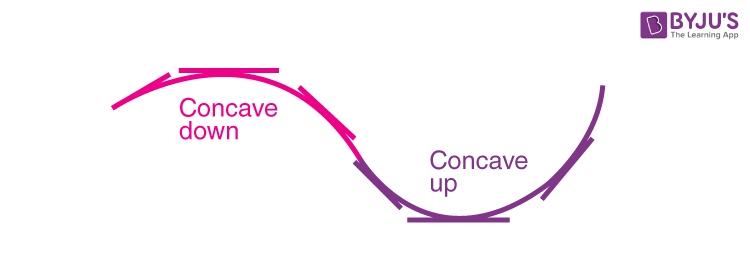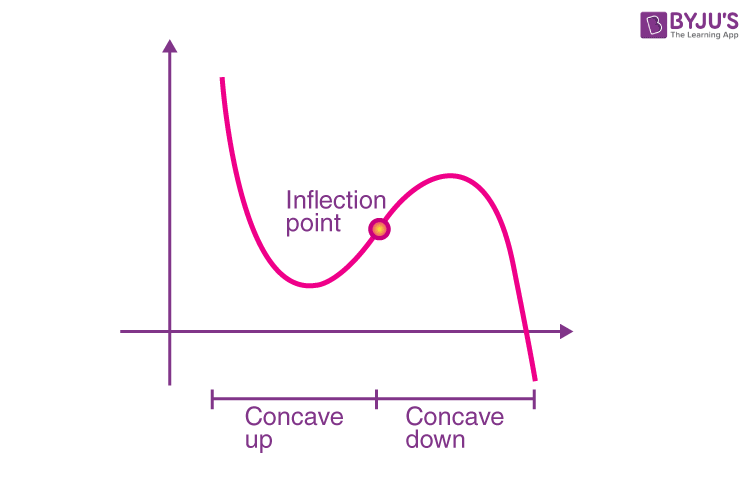# Inflection Point

In Mathematics, a function is a special relationship between two sets (input set and output set). Every member of the output set is uniquely related to one or more members of the input set. The function is represented by “f”. There are different types of functions. They are classified according to the categories. One such category is the nature of the graph. Depending upon the nature of the graph, the functions can be divided into two types namely

• Convex Function
• Concave Function

Both the concavity and convexity can occur in a function once or more than once. The point where the function is neither concave nor convex is known as inflection point or the point of inflection. In this article, the concept and meaning of inflection point, how to determine the inflection point graphically are explained in detail.

## Inflection Point Definition

The point of inflection or inflection point is a point in which the concavity of the function changes. It means that the function changes from concave down to concave up or vice versa. In other words, the point in which the rate of change of slope from increasing to decreasing manner or vice versa is known as an inflection point. Those points are certainly not local maxima or minima. They are stationary points.

## Concavity Function

Generally, when the curve of a function bends, it forms a concave shape. It is known as the concavity of a function. In graph function, two types of concavity can be found.

• Concave up
• Concave down

Concave Up – If a curve opens in an upward direction or it bends up to make a shape like a cup, it is said to be concave up or convex down.

Concave Down – If a curve bends down or resembles a cap, it is known as concave down or convex up. In other words, the tangent lies underneath the curve if the slope of the tangent increases by the increase in an independent variable.### Inflection Point Calculus

If f(x) is a differentiable function, then f(x) is said to be:

• Concave up a point x = a, iff f “(x) > 0 at a
• Concave down at a point x = a, iff f “(x) < 0 at a

Here, f “(x) is the second order derivative of the function f(x).

### Inflection Point GraphThe point of inflection defines the slope of a graph of a function in which the particular point is zero. The following graph shows the function has an inflection point.

It is noted that in a single curve or within the given interval of a function, there can be more than one point of inflection.

### How to Find the Inflection Point on a Graph?

An inflection point is defined as a point on the curve in which the concavity changes. (i.e) sign of the curvature changes. We know that if f ” > 0, then the function is concave up and if f ” < 0, then the function is concave down. If the function changes from positive to negative, or from negative to positive, at a specific point x = c, then that point is known as the point of inflection on a graph.

### Inflection Point of a Function

We can identify the inflection point of a function based on the sign of the second derivative of the given function.  Also, by considering the value of the first-order derivative of the function, the point inflection can be categorized into two types, as given below.

If f'(x) is equal to zero, then the point is a stationary point of inflection.

If f'(x) is not equal to zero, then the point is a non-stationary point of inflection.

### Inflection Point Examples

Refer to the following problem to understand the concept of an inflection point.

Example:

Determine the inflection point for the given function f(x) = x4 – 24x2+11

Solution:

Given function: f(x) = x4 – 24x2+11

The first derivative of the function is

f’(x) = 4x3 – 48x

The second derivative of the function is

f”(x) = 12x2 – 48

Set f”(x) = 0,

12x2 – 48 = 0

Divide by 12 on both sides, we get

x2 – 4 = 0

x2 = 4

Therefore, x = ± 2

To check or x = 2, substitute x= 1 and 3 in f”(x)

So, f”(1) = 12(1)2 – 48 = -36 (negative)

f”(3) = 12(3)2 – 48 = 276 (positive)

To check for x = -2, substitute x= 0 and -3 in f”(x)

So, f”(0) = 12(0)2 – 48 = -48 (negative)

f”(3) = 12(3)2 – 48 = 276 (positive)

Hence, proved

Now, substitute x = ± 2 in f”(x)

Therefore, it becomes

f”(2) = 12(2)2 – 48 = -69

f”(-2) = 12(-2)2 – 48 = -69

Therefore, the inflection points are (2, -69), and (-2, -69).

Register with BYJU’S – The Learning App for more Maths-related concepts. Also, watch interesting videos to learn with ease.

## Frequently Asked Questions on Inflection Point – FAQs

Q1

### What is an inflection point?

The point on a smooth plane curve at which the curvature changes sign is called an inflection point, point of inflection, flex, or inflection. In other words, it is a point in which the concavity of the function changes.
Q2

### How do you find a point of inflection?

The function f(x) is continuous and differentiable at a point x = a, has a second derivative f”(x) at a, in some deleted neighbourhood of the point x = a. If the sign of the second derivative of f(x) changes when passing through the point x = a, then x = a is a point of inflection of the given function f(x).
Q3

### Is an inflection point a turning point?

May or may not be since all the turning points are stationary, but not all the stationary points are turning points. A point at which the derivative of the function is zero, but its derivative’s sign does not change, identified as a point of inflection or saddle point.
Q4

### Where are inflection points on a graph?

The points on the graph of a function observed at the point where the curve changes its concavity that means from U to ∩ or vice versa.
Q5

### Can an inflection point be undefined?

As we know, a point of inflection is a point on the graph at which the graph’s concavity changes. If a function is undefined at a particular value of x, then there can be no inflection point. There is a possibility that the concavity can change as we move over the x value, from left to right, for which the function may not be undefined.